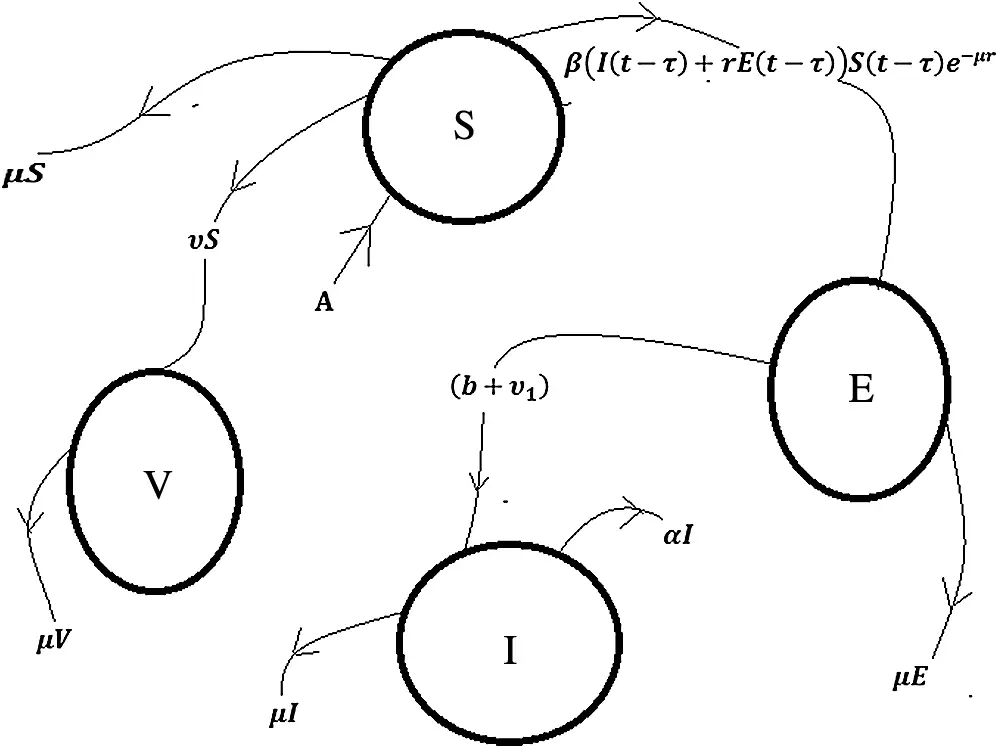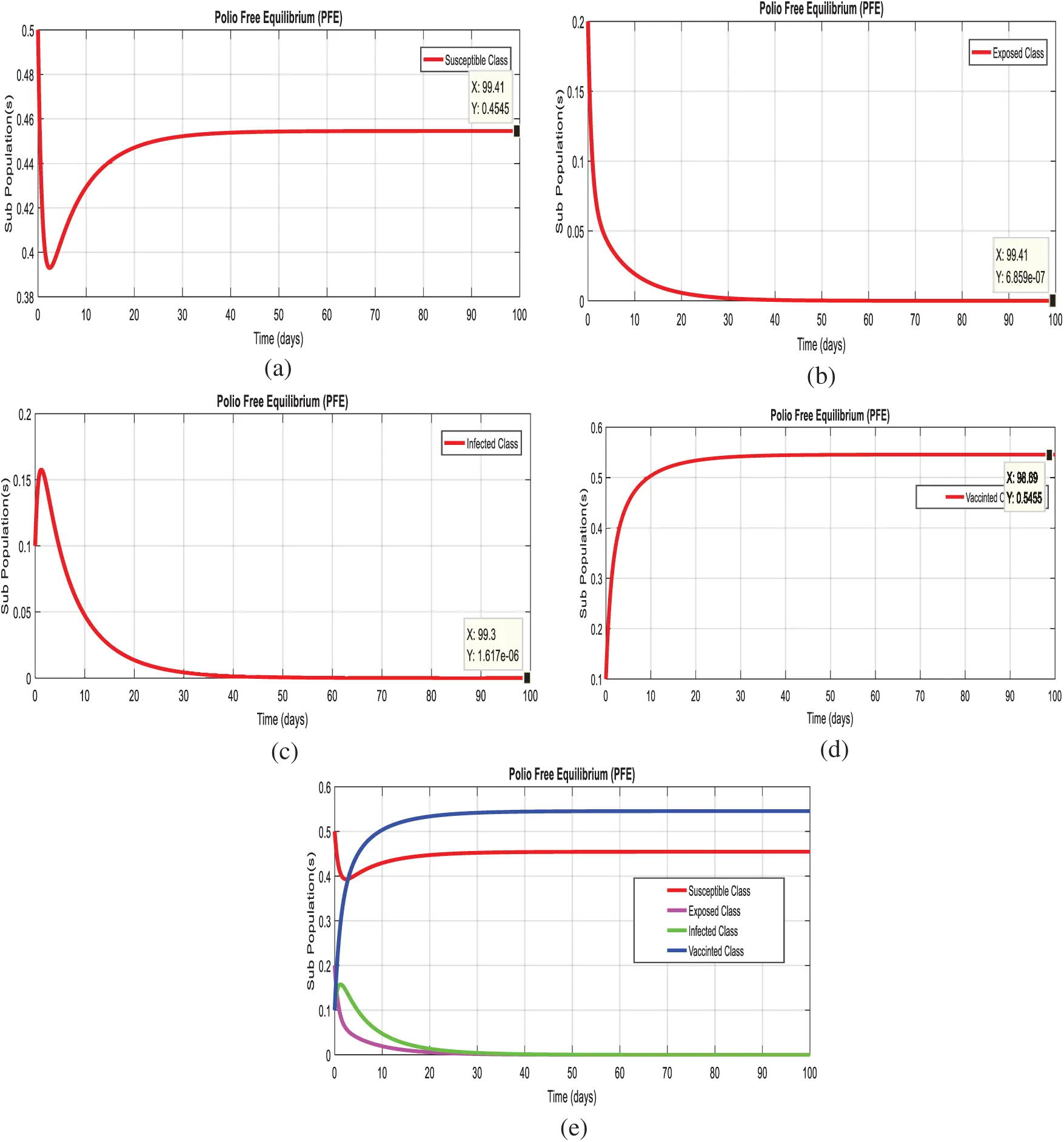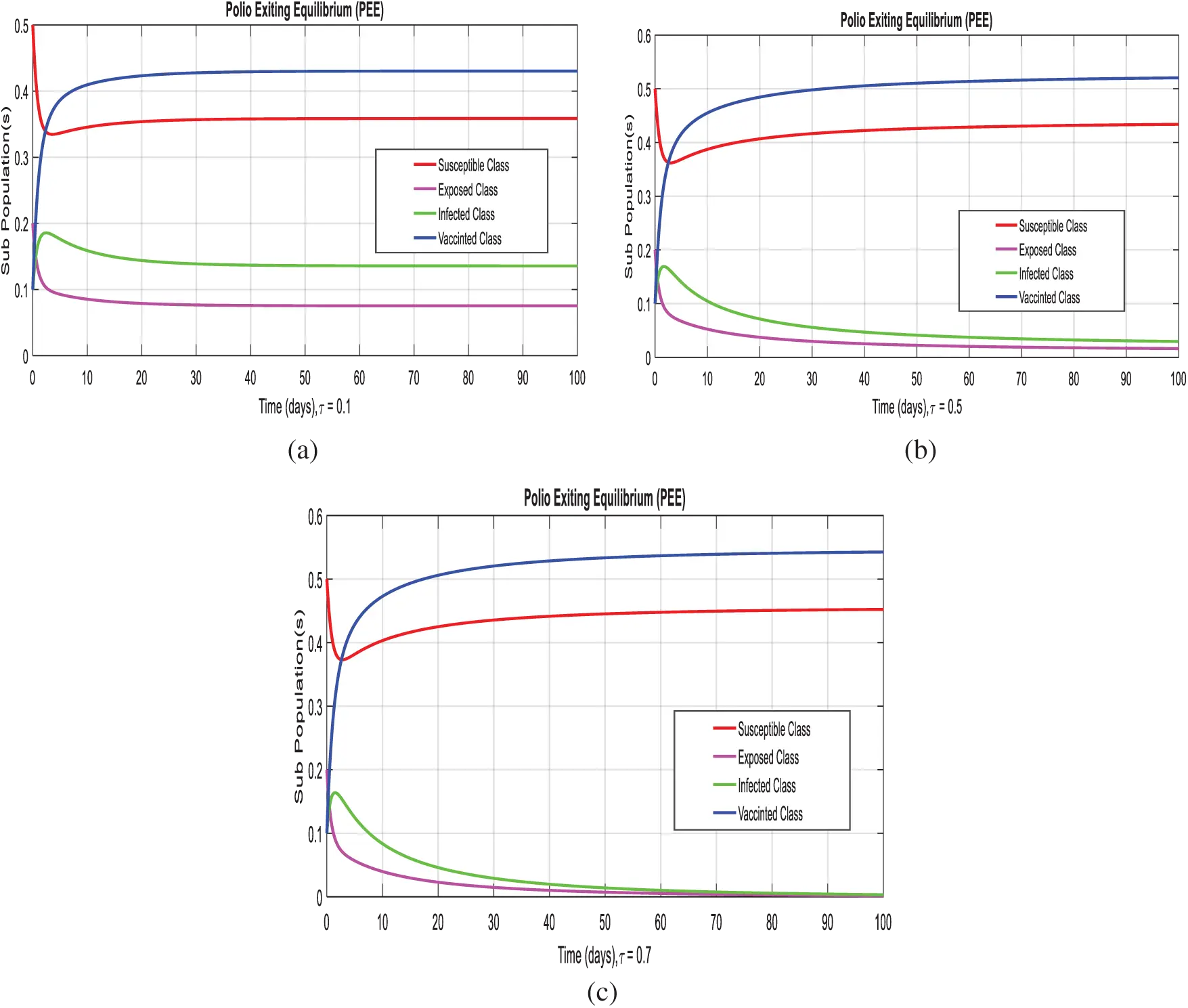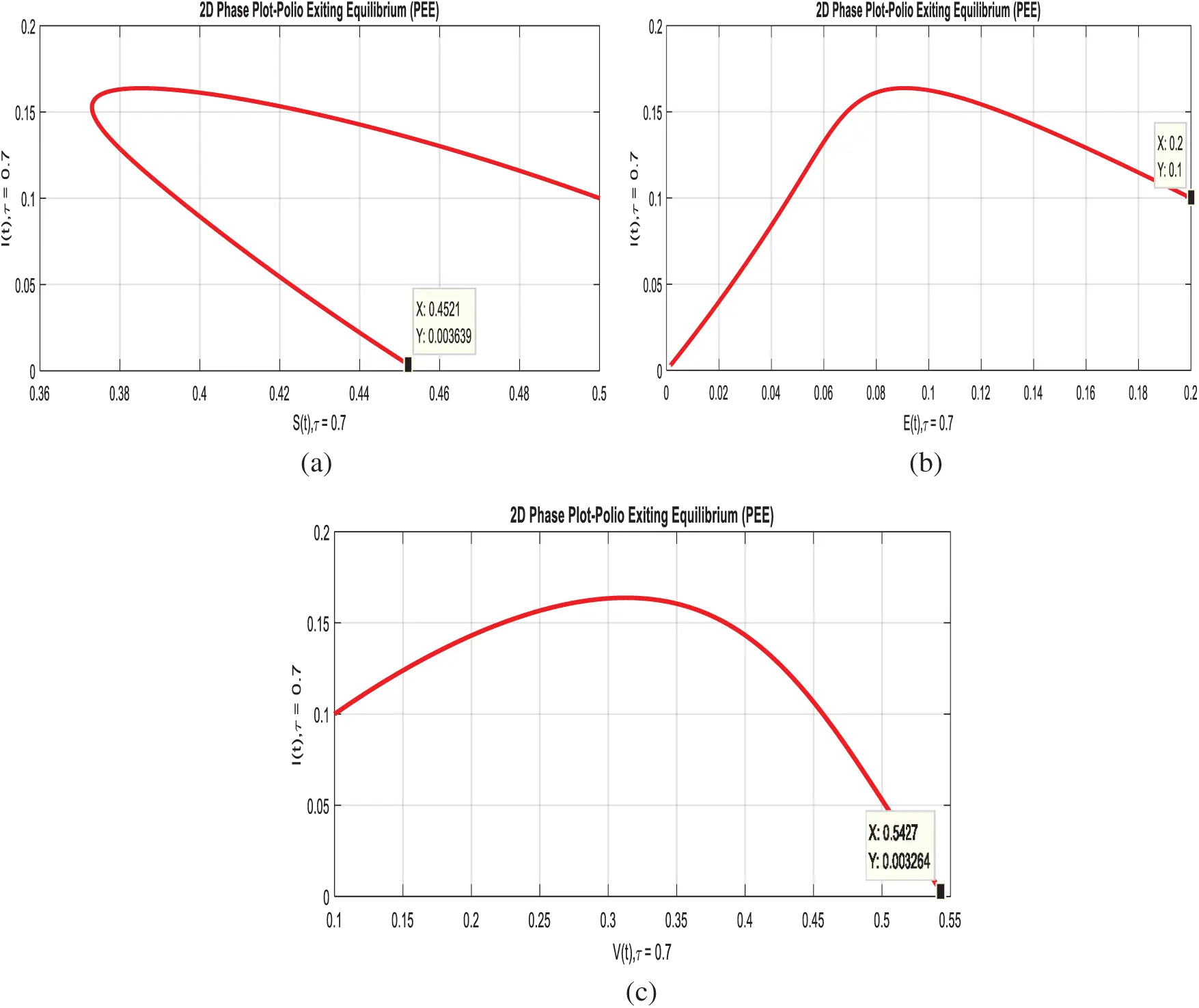[BACK]
 Computers, Materials & ContinuaDOI:10.32604/cmc.2022.020112Article

Treatment of Polio Delayed Epidemic Model via Computer Simulations

1Department of Mathematics, Air University Islamabad, Pakistan
2Department of Mathematics, Cankaya University, 06530, Balgat, Ankara, Turkey
3Department of Medical Research, China Medical University, Taichung, 40402, Taiwan
4Department of Mathematics, National College of Business Administration and Economics Lahore, Pakistan
5Department of Mathematics, Faculty of Sciences, University of Central Punjab, Lahore, Pakistan
*Corresponding Author: Muhammad Naveed. Email: nvdm4u@gmail.com
Received: 10 May 2021; Accepted: 27 June 2021

Abstract: Through the study, the nonlinear delayed modelling has vital significance in the different field of allied sciences like computational biology, computational chemistry, computational physics, computational economics and many more. Polio is a contagious viral illness that in its most severe form causes nerve injury leading to paralysis, difficulty breathing and sometimes death. In recent years, developing regions like Asia, Africa and sub-continents facing a dreadful situation of poliovirus. That is the reason we focus on the treatment of the polio epidemic model with different delay strategies in this article. Polio delayed epidemic model is categorized into four compartments like susceptible, exposed, infective and vaccinated classes. The equilibria, positivity, boundedness, and reproduction number are investigated. Also, the sensitivity of the parameters is analyzed. Well, known results like the Routh Hurwitz criterion and Lyapunov function stabilities are investigated for polio delayed epidemic model in the sense of local and global respectively. Furthermore, the computer simulations are presented with different traditions in the support of the analytical analysis of the polio delayed epidemic model.

Keywords: Polio disease; delayed model; stability analysis; computer simulations

1  Introduction

2  Formulation of Model

In order to analyze the spread of the polio disease more naturally polio delayed epidemic model is presented. In which we divided the whole population N(t) in to four compartments namely susceptible, exposed, infected and vaccinated denoted by S(t),E(t),I(t) and V(t) respectively.

The others parameters of the model are defined as, A denotes the human’s recruitment rate which is constant, β represents the transmission rate of infection because of the infected individuals, also rβ(0<r1) reprsents the infection transmission rate due to exposed class, in this term r is the reduction in the transmission of infection due to exposed class because in case of polio, although exposed population transmit the infection to others yet transmission of infection by them is less than those in infective class. v represents the rate of recruits in the susceptible class moving to class of vaccinated, also the exposed individulas are vaccinated at the rate of v1 due to having no symptoms of polio in them, b is the rate at which exposed population move to the infective class; μ and α are the natural and disease-induced death rates of the human population, respectively. Mathematically the polio delayed epidemic model is presented by the following system of delay differential equations and its transmission is presented in Fig. 1.

dSdt=AβS(tτ)I(tτ)eμτrβS(tτ)E(tτ)eμτ(μ+v)S(t),t0 τt (1)

dEdt=βS(tτ)I(tτ)eμτ+rβS(tτ)E(tτ)eμτ(b+μ+v1)E(t),t0 τt (2)

dIdt=(b+v1)E(t)(μ+α)I(t),t0 (3)

dVdt=vS(t)μV(t),t0 (4)Figure 1: Flow chart of the polio disease model

Subject to non-negative initial conditions S = S0 ≥ 0, E = E0 ≥ 0, I = I0 ≥ 0, R = R0 ≥ 0.

2.1 Positivity and Boundedness of Model

In this section, we will discuss the positivity and boundedness of the polio delayed epidemic model. For the sake of significant analysis of the model, all the variables S(t),E(t),I(t),V(t) must be non-negative. Therefore the attainable results of the polio delayed epidemic model remain positive and bounded for any time ,t0 τt in a feasible region.

B={(S,E,I,R)R+4:N(t)Aμ,S0,E0,I0,V0}.

For positivity and boundedness, we will use the following results.

Theorem: The solutions (S,E,I,V)ϵR+4 of the system (1–4) is positive at any time t0,τt with given non-negative initial conditions.

Proof: It is clear from the system (1–4) as follows:

dSdt|S=0=A0,dEdt|E=0=βSIeµτ0,dIdt|I=0=(b+v1)E0,dVdt|V=0=rS0

Which is the desired result.

Theorem: The solutions (S,E,I,V)ϵR+4 of the system (1–4) is bounded.

Proof: Consider the following function:

N(t)=S(t)+E(t)+I(t)+VS(t),dNdt=AμNαIAμN,dNdtAμN.

By the Gronwall’s inequality, we get

N(t)N(0)eμt+Aμ,t0 limtSupN(t)Aµ

Which shows that the solution of the system (1–4) is bounded and lies in the feasible region B.

2.2 Analysis of Model

Equilibria of the polio delayed model will be discussed in this section like polio trivial equilibrium point (PTEP0), polio free equilibrium point (PFEP1) and polio existing equilibrium point as follows:

P0=(S0,E0,I0,V0)=(0,0,0,0),P1=(S1,E1,I1,V1)=(Aμ+v,0,0,vAμ(μ+v)),and  P2=(S,E,I,V)WhereS=(b+μ+v1)(μ+α)[r(μ+α)+(b+v1)]βeμτE=[A(μ+r)S](α+μ)S[β(b+v1)eμτ+rβeμτ(α+μ)] I=(b+v1)(μ+α)[[A(μ+r)S](α+μ)[β(b+v1)eμτ+rβeμτ(α+μ)]S],V=vμS

2.3 Reproduction Number

The most important factor of the model is the reproduction number denoted by R0. Which has a vital role in the disease dynamics, change in its value can change the dynamics of disease. If the value of R0 is less than 1, then it represents the situation when disease has died out or control in the population and if the value of R0 is greater than 1, then it shows that the disease is spreading in the population. We have considered the infectious and recovered compartments as follows:

[EIV]=[rβSeμτβSeμτ0000000][EIV][(b+μ+v1)00(b+v1)(μ+α)000μ][EIV]

where, X=[rβSeμτβSeμτ0000000] and Y=[(b+μ+v1)00(b+v1)(μ+α)000μ]

Consider, XY1=[rβSeμτ(b+μ+v1)+βSeμτ(b+v1)(μ+α)(b+μ+v1)00000000].

XY1|P1=[rβAeμτ(b+μ+v1)(μ+v)+βAeμτ(b+v1)(μ+α)(b+μ+v1)(μ+v)00000000].

By next generation matrix method, it can be concluded that the spectrum radius of XY1|P1 will represent the reproduction number which is given by R0=rβAeμτ(b+μ+v1)(μ+v)+βAeμτ(b+v1)(μ+α)(b+μ+v1)(μ+v).

2.4 Analysis of Sensitivity of Parameters

Parameters used in the model have substantial role in the dynamics of the disease. In this section we present the sensitivity analysis of the parameters as follows:

Pr=R0R0rr=rR0×βAeμτ(μ+v)(μ+b+v1)>0, Similarly for other parameters, we have the following results.

Pβ=1,PA=1>0,Pb=bR0[βAeμτ(μ(r1)+αr)(b+μ+v1)2(μ+v)(μ+α)]<0,Pα=αR0[βAeμτ(b+v1)(μ+b+v1)(μ+v)(μ+α)2]<0, Pv=vR0[βAeμτ(b+μ+v1)(μ+v)2(r+b+v1μ+α)]<0,Pv1=v1R0[βAeμτ(μ(r1)+αr)(μ+v)(μ+b+v1)]<0, Pμ=μR0[(βAeμτ(b+v1v)(μ+v)2+βAeμτ(vbv1)(μ+b+v1)2)(r+b+v1μ+α)+(βAeμτ(μ+b+v1)(μ+v))(b+v1(μ+α)2)]<0,

From the above calculations it can be concluded that r,β,A are sensitive while the parameters b,α,v,v1,μ are not sensitive.

3  Local Stability

In this section local stability of the polio delayed epidemic model will be checked at the equilibrium points of the model using the following well-known results.

Theorem: The polio trivial equilibrium (PTE-P0), D0=(S0,E0,I0,R0)=(0,0,0,0) is locally asymptotical stable (LAS) if R0=1.

Proof: The Jacobean matrix of polio delayed epidemic model is given by

JP=[βIeμτrβEeμτ(μ+v)rβSeμτβSeμτ0βIeμτ+rβEeμτrβSeμτ(b+μ+v1)βSeμτ00(b+v1)(μ+α)0v00μ]

The Jacobean matrix evaluated at polio trivial equilibrium point (PTE−P0) is given by

JP|P0=[(μ+v)0000(b+μ+v1)000(b+v1)(μ+α)0v00μ]

By evaluating |JP|P0λI|=0, we have the negative Eigen values λ1=(μ+v)<0,λ2=μ and the polynomial λ2+a0λ+a1=0

where a0=(b+μ+v1)+(μ+α) and a1=(b+μ+v1)(μ+α) it can easily be observed that both a0, a1>0ifR0=1, so, by Routh-Hurwitz criterion the polio trivial equilibrium (PTEP0), is locally asymptotical stable.

Theorem: The polio free equilibrium, P1=(S1,E1,I1,V1)=(Aμ+v,0,0,vAμ(μ+v))is locally asymptotical stable (LAS) if R0<1. Otherwise, the system is unstable at P1 if R0>1.

Proof: The Jacobean matrix evaluated at polio free equilibrium point P1 is given by

JP1=[(μ+v)rβ(Aμ+v)Eeμτβ(Aμ+v)eμτ00rβ(Aμ+v)eμτ(b+μ+v1)β(Aμ+v)eμτ00(b+v1)(μ+α)0v00μ] |JP1λI|=|(μ+v)λrβ(Aμ+v)Eeμτβ(Aμ+v)eμτ00rβ(Aμ+v)eμτ(b+μ+v1)λβ(Aμ+v)eμτ00(b+v1)(μ+α)0v00μλ|=0

After solving the above determinant, the following Eigen values are found

λ1=(μ+v)<0, λ2=μ<0, also the polynomial λ2+n0λ+n1 = 0

where n0=(b+μ+v1)+(μ+α)rβ(Aμ+v)eμτ,

And n1=(b+μ+v1)(μ+α)rβ(Aμ+v)(μ+α)eμτ

So, by Routh-Hurwitz Criterion for 2nd degree polynomial, both fixed values of n0,n1>0  if R0<1. Hence the polio free equilibria (PFEP1) of the given system (1–4) is locally asymptotical stable.

Otherwise, if R0>1, then, Routh Hurwitz condition does not hold. Thus, P1 is locally asymptotical unstable.

Theorem: The polio existing equilibrium (PEE-P2), P2=(S,I,T,R) is locally asymptotical stable (LAS) if R0>1.

Proof: The Jacobean matrix of the system (1–4) at P2 is as follows:

JP2=[B1rB2(μ+v)rB3B30B1+rB2rB3(b+μ+v1)B300(b+v1)(μ+α)0v00μ]

where B1=βIeμτ, B2=βEeμτ, B3=βSeμτ

|JP2λI|=0λ1=μ<0, Also, we have the polynomial

λ3+g0λ2+g1λ+g2=0 g0=[(μ+v)(μ+α)rB2],g1=[rB3(μ+α)(μ+α)(μ+v)B1(μ+α)rB2(μ+α)rB3+(b+μ+v1)(μ+α)(b+μ+v1)+B3(b+v1)] and g2=[B1(b+μ+v1)B1Cr+ACr(μ+α)B1(μ+α)(b+μ+v1)+B1B3(b+v1)r2B2B3+rB2(b+μ+v1)+r2B2B3(μ+α)rB2(μ+α)(b+μ+v1)+rB2B3(b+v1)rB3(μ+v)+(μ+v)×(b+μ+v1)+rB3(μ+α)(μ+v)(μ+α)(μ+v)(b+μ+v1)B3(b+v1)]

So, by Routh-Hurwitz Criterion for 3rd degree polynomial, the following constraint has been verified if, R0>1.

g2,g0>0 and ,g0g1>g2.

Hence, the polio existing equilibria (PEE-P2) of the given system (1–4) is stable in the sense of locally.

4  Global Stability

Well known results are presented for the stability of polio delayed epidemic model in the sense of globally as follows:

Theorem: The polio trivial equilibrium (PTEP0), P0=(S0,E0,I0,V0)=(0,0,0,0) is globally asymptotical stable (GAS) if R0=1.

Proof: Define the Volterra Lyapunov function F:BR defined as

F=S+E+T+V, forall (S,E,T,V)ϵB,dFdt=dSdt+dEdt+dIdt+dVdt dFdt=AβSIeμτrβSEeμτ(μ+v)S+βSIeμτ+rβSEeμτ(b+μ+v1)E+(b+v1)E(μ+α)I+vSμV dFdt=AμNαI. dFdtAμN.

dFdt0, if R0=1 and dFdt=0. Hence P0 is globally asymptotically stable.

Theorem: The polio free equilibrium (PFEP1), P1=(S1,I1,T1,R1)=(λμ,0,0,vμS0) is globally asymptotical stable (GAS), if R0<1.

Proof: Define the Volterra Lyapunov function F:BR defined as

F=(SS1S1logSS1)+E+I+(VV1V1logVV1),(S,I,E,V)ϵB dFdt=ddS(SS1S1logSS1)dSdt+dEdt+dIdt+ddV(VV1V1logVV1)dVdt dFdt=(1S1S)dSdt+dEdt+dIdt+(1V1V)dVdt dFdt=(SS1S)dSdt+dEdt+dIdt+(VV1V)dVdt dFdt=(SS1S)(AβSIeμτrβSEeμτμSvS)+(βSIeμτ+rβSEeμτbEμEv1E+bE+v1EμIαI)+(VV1V)(vSμV). dFdt=(SS1)(ASβSIeμτrβSEeμτμv)+[βSI+rβSE]eμτμEμIαI+(VV1)(vSVμ). dFdt=(SS1)(ASAS1βIeμτrβEeμτvμI+βIeμτμE+rβEeμτ+v)+βISeμτ+rβSeμταI+(VV1)(vSVvS1V1). dFdt=(SS1)(1S1S1)AμE(1rβSeμτμ)μI(1βSeμτμ)αI+(VV1)(1V1v0)vS. dFdt=(SS0)2SS0AμE(1rβSeμτμ)μI(1βSeμτμ)αI(VV1)2VV1vS.

From the above calculations, it can be observed that dFdt<0, if R0<1 and dFdt=0 if S=S1,I=0,E=0andV=V1. Hence P0 is globally asymptotically stable.

Theorem: The polio existing equilibrium (PEEP), P=(S,I,E,V) is globally asymptotical stable (GAS), if R0>1.

Proof: Considering the Volterra Lyapunov function F:BR defined as

F=(SSSlogSS)+(EEElogEE)+(IIIlogII)+(VVVlogVV) dFdt=ddS(SSSlogSS)dSdt+ddE(EEElogEE)dEdt+ddI(IIIlogII)dIdt+dVdt(VVVlogVV)dVdt. dFdt=(SSS)(AβSIeμτrβSEeμτ(μ+v)S)+(EEE)(βSIeμτ+rβSEeμτ(bE+μE+v1E))+(III)((bE+v1E)(μI+αI))+(VVV)(vSμV). =(SS)(ASβIeμτrβEeμτ(μ+v))+(EE)(βSIeμτE+rβSeμτ(b+μ+v1))+(II)((bEI+v1EI)(μ+α))+(VV)(vSVμ) dFdt=(SS)[ASASβIeμτ+βIeμτrβEeμτ+rβEeμτv+v]+(EE)[βSIeμτEβSIeμτE+rβSeμτrβSeμτb+bv1+v1]+(II)[bEIbEI+v1EIv1EIα+α]+(VV)(VVVV)vS. dFdt=(SS)2(SS)A(EE)2βSIeμτ(EE)(II)2(II)bE(II)2(II)βeμτ(VV)2(VV)rS.

It can be concluded from the above equation that dFdt0 for R0<1, also dFdt=0 only if S=S,I=I,E=E and V=V. Hence P is globally asymptotically stable (GAS).

5  Computer Simulation

In this section, the numerical treatments of polio delayed epidemic model are presented by using the values of the parameters as discussed in Tab. 1 as follows :Example 1: (Simulation at polio free equilibrium (PFEP1) without delay).

Figs. 2a2d, displays the solution of the system (1–4), at the polio free equilibrium (PFEP1), P1=(S1,E1,I1,V1)=(0.4545,0,0,0.5455) with the initial data of the model S(0)=0.5, E(0)=0.2, T(0)=0.1, V(0)=0.1 and the fixed values of the model presented in Tab. 1. Therefore, the system (1–4) touches to P1, in the absence of delay the value of reproduction number is R0 = 0.7482 < 1. Moreover, Fig. 2e, shows combine behavior of the system (1–4) without consideration of the delay effect, as desired.Figure 2: Time plots of the system (1–4) at the polio free equilibrium of model. For any time t (a) Susceptible class (b) Exposed class (c) Infected Class (d) Vaccinated class (e) Combined behavior of each sub-population(s)

Example 2: (Simulation at polio existing equilibrium (PEEP2) without delay)

Figs. 3a3d, displays the solution of the system (1–4), at the diarrhea existing equilibrium (PEEP2), P2=(S,E,I,V)=(0.3411,0.0895,0.1604,0.4094), Therefore, the system (1–4) converges to P2, in the absence of delay the value of reproduction number is R0 = 1.4950 > 1. Moreover, Fig. 3e, shows combine behavior of the system (1-4) without consideration of the delay effect, as desiredFigure 3: Time plots of the system (1–4) at the Polio existing equilibrium of model. For any time t (a) Susceptible class (b) Exposed class (c) Infected Class (d) Vaccinated class (e) Combined behavior of each sub-population(s)

Example 3: (Simulations at polio existing equilibrium with time delay effect)

In this section, we taken the effect of the system (1–4), at the diarrhea existing equilibrium of the model with effective use of artificial delay tactics. Figs. 4a4c, we can observe the susceptibility of humans increases with use of delay tactics. In the other hand, we can observe the infectivity of polio patient decreases and even converges to zero.Figure 4: Time plots of the system (1–4) at the polio existing equilibrium with the effective uses of delay tactics. (a) Combined behavior at τ=0.1 (b) Combined behavior at τ=0.5 (c) Combined behavior at τ=0.7

Example 4: (Effectiveness of delay tactics on the reproduction number of the model).

Let, τ=1.011. As clear, the reproduction value decreases that moves the dynamics of the system of polio virus moves from existing scenario to polio free equilibrium. Yet, Fig. 5 displays the fact that the increases in delay strategy can overcome the infectivity of polio viruses, as needed.Figure 5: Comparison graph of the reproduction number and artificial delay term of the model

Example 5: (Replication for the consequence of delay term on the infective class).

For the different values of τ, exhibits that infective class of peoples reduces even completely die out from population. Subsequently, Fig. 6 displays the fact that the delay strategies such as vaccinations in different age groups, as desired.Figure 6: Display of the effect of delay term on infective class of the model

Example 6: (2D-simulations of the system with delay effect).

In this section, we plotted two-dimensional behavior of the model with the effective uses of artificial delay techniques. Even though, theses simulations are the interaction of susceptible class with remaining compartments of the model. Figs. 7a7c, exhibits that immunity of human’s dominant with the immunity of infected humans, as desired.Figure 7: 2-D phases of the system (1–4) with delay effect. (a) Susceptible and infected classes at τ=0.7 (b) Infected and exposed classes at τ=0.7 (c) Vaccinated and infected classes at τ=0.7

6  Conclusion

In this article, we investigated the dynamics of the polio delayed epidemic model with the effective use of delay strategies. In which we divided the entire population into four different groups which are susceptible, exposed, infected and vaccinated. We have calculated the reproduction number for the polio delayed epidemic model and also gave the sensitivity analysis of the parameters involved in reproduction number. We have also presented the local and global stabilities of the model at the equilibrium of the model, that is, at polio-free equilibrium and at polio existing equilibrium by using well-known results of mathematics. In addition, we carefully studied the effect of delay factor on infected class, exposed class and reproduction number and we have come to the conclusion that we can control the dynamics of polio by using different effected techniques of delay like vaccination is proved to be the ultimate solution to avoid the spread of this disease. Immunization is much important and is recommended for children’s who fall under the age group of 2 to 18 months. Moreover, Booster doses are recommended for children’s up to 12 years of age to make sure of immunization. And most importantly a good hygiene system at a personal level is much needed to adapt, which can play a vital role to reduce the spread of the poliovirus.

Funding Statement: The authors received no specific funding for this study.

Conflicts of Interest: The authors declare that they have no conflicts of interest to report regarding the present study.

## References

1. D. M. Emperador, D. E. Velasquez, C. F. Estivariz, B. Lopman, B. Jiang et al., “Interference of monovalent, bivalent, and trivalent oral poliovirus vaccines on monovalent rotavirus vaccine immunogenicity in rural Bangladesh,” Clinical Infectuious Diseases, vol. 62, no. 1, pp. 150–156, 2015.
2. R. Haque, C. Snider, Y. Liu, J. Z. Ma, L. Liu et al., “Oral polio vaccine response in breastfed infants with malnutrition and diarrhea,” Vaccine, vol. 32, no. 4, pp. 478–482, 2014.
3. M. Taniuchi, J. A. Platts-Mills, S. Begum, M. J. Uddin, S. U. Sobuz et al., “Impact of enterovirus and other enteric pathogens on oral polio and rotavirus vaccine performance in Bangladeshi infants,” Vaccine, vol. 34, no. 27, pp. 3068–3075, 2016.
4. H. Manukyan, T. Zagorodnyaya, R. Ruttimann, Y. Manor, A. Bandyopadhyay et al., “Quantitative multiplex one-step RT-PCR assay for identification and quantitation of Sabin strains of poliovirus in clinical and environmental specimens,” Journal of Virological Methods, vol. 259, no. 1, pp. 74–80, 2018.
5. G. R. Macklin, O. Reilly, K. M. Grassly, N. C. Edmunds, W. J. Mach et al., “Evolving epidemiology of poliovirus serotype 2 following the withdrawal of the serotype 2 oral poliovirus vaccine,” Science, vol. 368, no. 6489, pp. 401–405, 2020.
6. K. M. Thompson and D. A. Kalkowska, “Review of poliovirus modeling performed from 2000 to 2019 to support global polio eradication,” Expert Review of Vaccines, vol. 7, no. 19, pp. 661–686, 2020.
7. A. A. Hamedan, M. Elaziz, P. Jiao, A. H. Alavi, M. Bahgat et al., “Prediction of the vaccine-derived poliovirus outbreak incidence: A hybrid machine learning approach,” Scientific Reports, vol. 10, no. 5058, pp. 1–12, 2020.
8. G. R. Macklin, M. T. Yeh, E. Bujaki, P. T. Dolan, M. Smith et al., “Engineering the live-attenuated polio vaccine to prevent reversion to virulence,” Cell Host and Microbe, vol. 28, no. 5, pp. 736–751, 2020.
9. O. K. M. Reilly, N. C. Grassly, W. J. Edmunds, O. Mach, R. S. G. Krishnan et al., “Evolving epidemiology of poliovirus serotype 2 following the withdrawal of the serotype 2 oral poliovirus vaccine,” Science, vol. 368, no. 6489, pp. 401–405, 2020.
10. M. Famulare, W. Wong, R. Haque, M. J. A. Platts, P. Saha et al., “Community structure mediates Sabin 2 polio vaccine virus transmission,” MedRxiv, vol. 1, no. 1, pp. 1–20, 2020.
11. D. A. Kalkowska, M. A. Pallansch, A. Wilkinson, A. S. Bandyopadhyay, A. J. L. Konopka et al., “Updated characterization of outbreak response strategies for 2019--2029: Impacts of using a novel type 2 oral poliovirus vaccine strain,” Risk Analysis, vol. 41, no. 2, pp. 329–348, 2020.
12. M. O. Kolade and A. Abdon, “Mathematical analysis and computational experiments for an epidemic system with nonlocal and nonsingular derivatives,” Chaos, Solitons & Fractals, vol. 1, no. 126, pp. 41–49, 2019.
13. R. J. Tebbens Duintjer and K. M. Thompson, “Polio endgame risks and the possibility of restarting the use of oral poliovirus vaccine,” Expert Review of Vaccines, vol. 17, no. 8, pp. 739–751, 2018.
14. R. J. Tebbens Duintjer and K. M. Thompson, “Poliovirus vaccination during the endgame: Insights from integrated modeling,” Expert Review of Vaccines, vol. 16, no. 6, pp. 577–586, 2017.
15. R. D. Tebbens and K. M. Thompson, “Comprehensive screening for immunodeficiency-associated vaccine-derived poliovirus: An essential oral poliovirus vaccine cessation risk management strategy,” Epidemiology and Infection, vol. 145, no. 02, pp. 217–226, 2017.
16. K. Zhao, J. Jorba, J. Shaw, J. Iber, O. Chen et al., “Are circulating type 2 vaccine-derived polioviruses (VDPVs) genetically distinguishable from immunodeficiency-associated VDPVs,” Computational and Structural Biotechnology Journal, vol. 15, no. 1, pp. 456–462, 2017.
17. M. Taniuchi, M. Famulare, K. Zaman, M. J. Uddin, B. A. M. Upfill et al., “Community transmission of type 2 poliovirus after cessation of trivalent oral polio vaccine in Bangladesh: An open-label cluster-randomized trial and modelling study,” The Lancet Infectious Diseases, vol. 17, no. 10, pp. 1069–1079, 20
18. M. Taniuchi, M. J. A. Platts, S. Begum, M. J. Uddi, S. U. Sobuz et al., “Impact of enterovirus and other enteric pathogens on oral polio and rotavirus vaccine performance in Bangladeshi infants,” Vaccine, vol. 34, no. 27, pp. 3068–3075, 2016.
19. R. J. D. Tebbens, L. M. Hampton, S. G. Wassilak, M. A. Pallansch, S. L. Cochi et al., “Maintenance and intensification of bivalent oral poliovirus vaccine use before its coordinated global cessation,” Journal of Vaccines and Vaccination, vol. 7, no. 5, pp. 3068–3075, 2016.
20. D. M. Emperador, D. E. Velasquez, C. F. Estivariz, B. Lopman, B. Jiang et al., “Interference of monovalent, bivalent, and trivalent oral poliovirus vaccines on monovalent rotavirus vaccine immunogenicity in rural Bangladesh,” Clinical Infectious Diseases, vol. 62, no. 2, pp. 150–156, 2015.
21. R. Haque, C. Snider, Y. Liu, J. Z. Ma, L. Liu et al., “Oral polio vaccine response in breastfed infants with malnutrition and diarrhea,” Vaccine, vol. 32, no. 4, pp. 478–482, 2014.
22. K. M. Thompson and D. R. J. Tebbens, “Current polio global eradication and control policy options: Perspectives from modeling and prerequisites for oral poliovirus vaccine cessation,” Expert Review of Vaccines, vol. 11, no. 4, pp. 449–459, 2012.
23. N. C. Grassly, C. Fraser, J. Wenger, J. M. Deshpande, R. W. Sutter et al., “New strategies for the elimination of polio from India,” Science, vol. 314, no. 5802, pp. 1150–1153, 2006.
24. M. Agarwal and A. S. Bhadauria, “Modeling spread of polio with the role of vaccination,” Applications and Applied Mathematics: An International Journal, vol. 6, no. 2, pp. 552–571, 2011.This work is licensed under a Creative Commons Attribution 4.0 International License, which permits unrestricted use, distribution, and reproduction in any medium, provided the original work is properly cited.